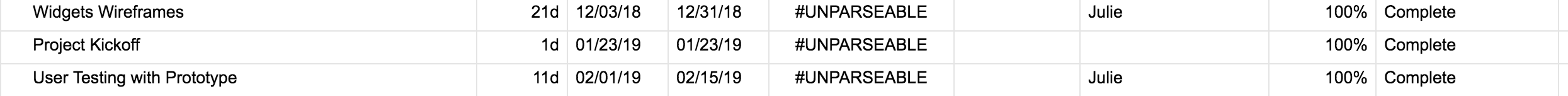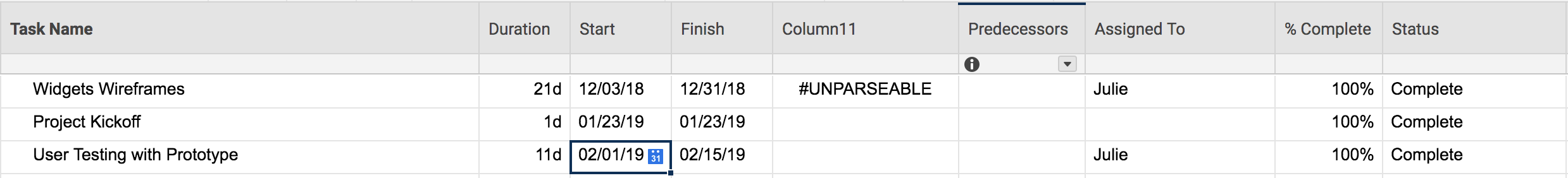# RYGB #unparseable

I have tried multiple formula listed on the community to automate the RYG column and i keep getting the unparseable error. I just need a formula to be able to show a red ball if the finish date has passed, Green if it completed, yellow if it is in progress and it is today.

• Can you provide your current formula along with more details such as your column names or possibly a screenshot with sensitive/confidential data hidden?

• =IF(STATUS@row = "Completed"; "Green"; IF(AND(STATUS@row = "In Progress"; [Start Date]@row < TODAY(); [Finish Date]@row > TODAY()); "Yellow"; IF(OR(AND(STATUS@row = "Not Started"); AND([Start Date]@row < TODAY(); OR(AND(STATUS@row = "In Progress"; [Finish Date]@row < TODAY())))); "Red")))• Try something like this...

=IF(Status@row = "Complete"; "Green"; IF([Finish Date]@row < TODAY(); "Red"; "Yellow"))

• I still get the unparseable error• Well.... without you posting how you're actual formula looks like now it's difficult ;-)

Anyway:

- did you make sure, that the column names exactly match the names in your formula and that "% Complete" has [ ] around it (and "Task Name")?

- if your account is set to english, then you must use comma, not ; !

Hope that helps.

Projektmanagement Professional in Frankfurt am Main, Germany

• I was running into this too, and my spectacular jira admin came up with this formula for me.

I now no longer get the unparseable error. It is strictly dependent on your due date column.

Use Case:

If Complete = Blue

If Due date is in the past = Red

If Due date is in the future = Green

If Due date is today (give or take 2 days) = Yellow

Formula:

=IF(Status@row = "Complete", "Blue", IF([Due Date]@row < TODAY(), "Red", IF([Due Date]@row = TODAY(-2), "Yellow", "Green")))

Hope this helps!

• Amanda,

This is definitely a good solution. It is the same concept as the one I posted above, but this one uses the fourth color. Well done.• =IF(Status@row = "Complete"; "Green"; IF(Finish@row < TODAY(); "Red"; "Yellow"))

Here it is updated for your column names.

• Hello, I am trying to use the Red, Green, Yellow and Gray balls but I am having issues. Please see my formula below.

=IF(ISBLANK([Due Date]@row), "", IF([Due Date]@row > TODAY(3), "Green", IF(AND([Due Date]@row > TODAY(), [Due Date]@row < TODAY(3)), "Yellow", IF([Due Date]@row <= TODAY(), "Red", IF(Status@row = "Complete"; "Gray"))))

it works if I remove: , IF(Status@row = "Complete"; "Gray"

But I need the Gray ball

• Swap the semicolon for a comma.

I also suggest putting the "Gray" section first. That way it will remain Gray even after the due date has passed since it is complete.

• It worked.....Thank you!